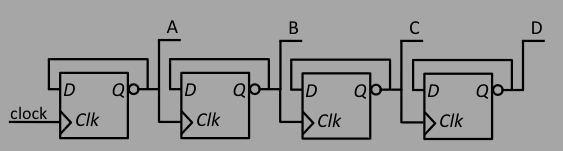## Assignments

Consider the truth table:
xyzF
0001
0011
0100
0111
1000
1010
1100
1111
1. Use SOP to calculate the boolean solution to F
2. Use the axioms of boolean algebra to simplify
3. Do the same thing with POS

1. Convert the base 10 (decimal) number 2913 to binary
2. Convert the binary number from above to a hexadecimal number

A "2-bit adder" is something that addes 2 numbers together, each of which has 2 bits. Let the inputs be A[1:0] = {A1,A0} and B[1:0] = {B1,B0}. The {} notation is called a "concatenation". The output will be a 2-bit sum S[1:0] = {S1,S0} and a carry bit C.
1. Construct the truth table for the inputs and outputs
2. Calculate using either SOP or POS the network you would need to build to implement this.

We know how to make a "count-down" counter using a clock and 4 bits, where the counter changes its output ever clock cycle. It looks like this:How would you construct a count-up counter?

Now that we know how to program the FPGA, latch data from the bit switches, and display data, we can make an FPGA calculator. We will need to attach all 16 bit switches, LEDs, push buttons, and the 4 digit display. Make a simple calculator that uses pushbuttons for: reset; add; subtract; execute operation. Then use the 16 bit switches for the input operands, and use one of the LEDs for the "sign" (as in plus/minus for the subtraction).

For added credit, use another pushbutton to transmit 2 bytes to a Python application running on the PC, and make a real calculator.

For another additional credit, write a Python app that will take the place of the push buttons, and invent a protocol where the computer sends instructions to the FPGA, and the FPGA executes and sends back data.

For the PWM, modify the triangle to make a ramp, and a sine wave, and use the push buttons to tell which one. For sine wave:
• /PWM/pwm_wavegen2
• switch 15 to start, 14 for ramp, 13 for triangle, 12 for sine
• use C=$1\mu F$ and R=$300\Omega$ to get a sine wave of $\sim 12 Hz$